# 1.0 Introduction

Equalizers are devices used to compensate for the negative gain slope of amplifiers. Unlike a standard attenuator with a flat frequency response, an equalizer is a unique kind of attenuator which exhibits lower insertion loss as frequency increases with some known slope.  This is a useful characteristic for system designers working with wideband amplifiers.  In this application note, we explore how equalizers are used to compensate for negative gain slope in various amplifier applications.  The different types of equalizers and their various characteristics are reviewed, and a case study is presented pairing Mini-Circuits’ PHA-1+ wideband MMIC amplifier with MMIC equalizer EQY-6-63+.  Test data is provided to illustrate the combined response.

# 2.0   Managing Negative Gain Slope

Most general purpose MMIC amplifiers operate over wide bandwidths, covering multiple application bands.  However, such amplifiers often exhibit a gain response that decreases with frequency.  This is especially true for wideband amplifiers with high gain.  Negative gain slope can be a major limitation for broadband applications which require consistent gain performance across wide frequency ranges.  Undesirable slope in the gain vs. frequency response may be exacerbated by cascading such amplifiers in series, a common technique to increase overall gain.

One way to get around the problem is to use different amplifiers for different frequency bands with specific gain values in narrow bandwidths.  However, this approach adds cost and component count to a design, and occupies more space on the user’s PCB layout.  These factors can make this piecewise approach impractical, and it is usually preferable to use one device with flat gain over a wide frequency band.  This is where equalizers come in.  Adding an equalizer at the output of a wideband amplifier creates a gain-flattening effect by combining the gain response of the amplifier with the insertion loss response of the equalizer.  The tradeoff is that some gain will be sacrificed for flatness over a wider usable bandwidth.

# 3.0 The Different Types of Equalizers

There are four basic types of equalizers available, each of which may be appropriate depending on the specific application and the amplifier being used:

• Positive Slope Equalizers (Figure 1) exhibit an insertion loss response with a positive slope vs. frequency. This type of equalizer is used to equalize amplifiers or systems with positive gain slope.
• Negative Slope Equalizers (Figure 2) exhibit an insertion loss response that decreases with frequency. This type of equalizer is used to equalize amplifiers or systems with negative gain slope, as in the example below.
• Positive Parabolic Equalizers (Figure 3) have an insertion loss response that increases in a parabolic shape as frequency increases. These are used to equalize gain variation in systems that have high gain at the band edges and low gain near the center frequency.
• Negative Parabolic Equalizers (Figure 4) exhibit insertion loss that decreases in a parabolic shape as frequency increases, and are used to equalize gain variation in systems that have low gain at the band edges but high gain at mid-band
 Figure 1: Positive slope equalizer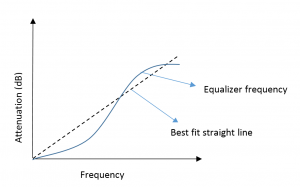Figure 2: Negative slope equalizer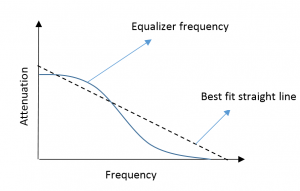Figure 3: Positive parabolic equalizer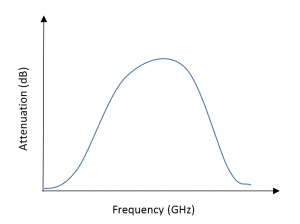Figure 4: Negative parabolic equalizer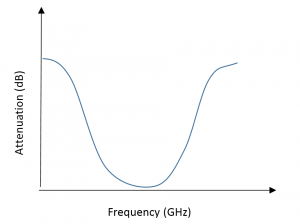# 4.0 Case Study: Pairing PHA-1+ MMIC Amplifier with EQY-6-63+ Negative Fixed Slope Equalizer

To demonstrate how equalizers can be used to produce a flat gain response from wideband amplifiers, in this example we pair the PHA-1+ high-dynamic range MMIC amplifier with the EQY-6-63+ MMIC fixed equalizer.  Mini-Circuits’ EQY-series of MMIC negative fixed slope equalizers is available in a range of dB slopes from 1 to 10 dB, allowing users to choose the proper value for the desired combined response with their amplifier.  Responses of models EQY-2-63+ (Figure 5) and EQY-6-63+ (Figure 6) shown below exhibit 2 dB and 6 dB slopes, respectively.  Additional benefits of these models are the excellent return loss (20 dB typ.), outstanding power handling (+31 dBm typ.) and small package size (2 x 2mm).

 Figure 5: Insertion loss response for EQY-2+ MMIC equalizer with –2 dB slope.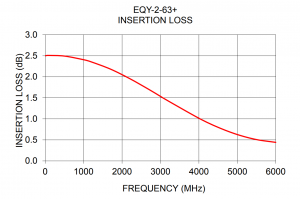Figure 6: Insertion loss response for EQY-6+ MMIC equalizer with -6 dB slope.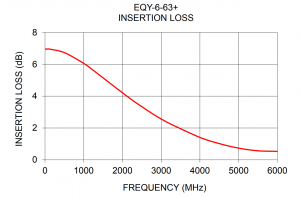The PHA-1+ amplifier boasts an operating frequency range from 0.5 to 6 GHz, but its gain varies from 16.5 dB at 100 MHz to 10 dB at 6 GHz.  The EQY-6-63+ equalizer model has an insertion loss varying from 7 dB at 100 MHz to 0.5 dB at 6 GHz, which makes it an excellent companion for the negative gain slope of the amplifier.  We expect the combined response to equalize the gain of the PHA-1+ to a flat value of around 9.5 to 10 dB.

The test setup shown in Figure 7 was used to test the combined response of the amplifier and equalizer together. Evaluation boards for each unit were cascaded in series with the equalizer at the amplifier output and connected to a vector network analyzer.  Gain and Return Loss were swept over frequency.  To counter any variation in testing, 5 units of PHA-1+ and 3 units of EQY-6-63+ were taken into account.  The eval boards for the amplifier and equalizer are shown in Figures 8a and 8b and are available from stock for customers’ testing needs.Figure 7: Test setup for PHA-1+ and EQY-6-63+

 Figure 8a: Evaluation board for PHA-1+ MMIC Amplifier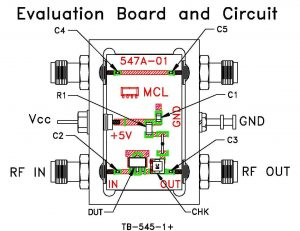Figure 8a: Evaluation board for EQY-6-63+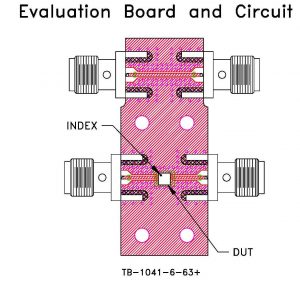# 5.0 Test Data

Figures 7 and 8 show the measured insertion loss response of the EQY-6-63+ equalizer with a negative slope of about 6 dB and the gain response of the PHA-1+ amplifier, also with a negative slope of roughly 6 dB.  We expect the higher loss at the low end of the equalizer response to flatten the higher gain at the low end of the amplifier response, resulting in flatter overall performance over the 0.5 to 6 GHz range.

 Figure 9: Negative insertion loss slope (6 dB) for EQY-6-63+ MMIC equalizer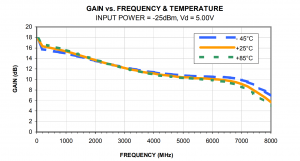Figure 10: Negative gain slope response for PHA-1+ MMIC amplifier, exhibiting 12 dB gain at mid-band and ±4 dB variation

Figure 9 shows the measured frequency response PHA-1+ and EQY-6-63+ combined in series on the test board.   Note the flat response with mid-band gain of 10 dB and minimal variation of +/-0.6dB across the entire 0.5 to 6 GHz range.  This result bears out the expected effect based on the individual responses of the amplifier and equalizer.Figure 11: Combined response of PHA-1+ and EQY-6-63+ in series.

Figures 10 and 11 illustrate that the input and output return loss of the cascaded pair are better than or equal to that of the amplifier when used alone.  This is due to the excellent return loss of the EQY-6-63+ and great matching over the full bandwidth.  The plots in Figure 12 show a comparison of the gain response of the amplifier alone vs. the amplifier-equalizer pair, effectively compensating for the negative gain slope of the amplifier.

 Figure 12: Comparison of input return loss for amplifier alone vs. amplifier-equalizer combination.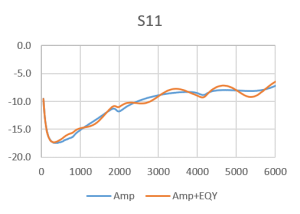Figure 13: Comparison of output return loss for amplifier alone vs. amplifier-equalizer combination.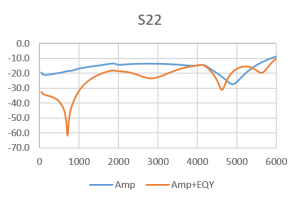Figure 14: Comparison of gain response for amplifier alone vs. amplifier equalizer combination.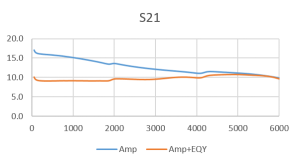# 6.0 Conclusion

Among all units tested, average flatness of equalized gain fell within ±1.3 dB.  The PHA-1+ has a gain variation of 6 dB from 100 MHz-6000 MHz.  By using the EQY-6-63+ in series with the PHA-1+, we were able to compensate for the gain variation with the negative 6 dB insertion loss slope of the equalizer.  The EQY-6-63+ is therefore an excellent match to be used alongside the PHA-1+ amplifier in order to obtain a wideband, flat gain response.  This is just one example of how amplifier-equalizer pairs can be used to flatten gain response when using wideband amplifiers.

# 6.0 Other Recommended Amplifier-Equalizer Pairs

As an additional example of matches that lead to optimal results, we recommend the following:

 Amplifier Equalizer GVA-62+ EQY-2-63+ GALI-19+ EQY-2-63+ GVA-81+ EQY-3-63+ GVA-63+ EQY-5-63+ PGA-102+ EQY-6-63+ GVA-83+ EQY-8-63+ CMA-84+ EQY-10-63+